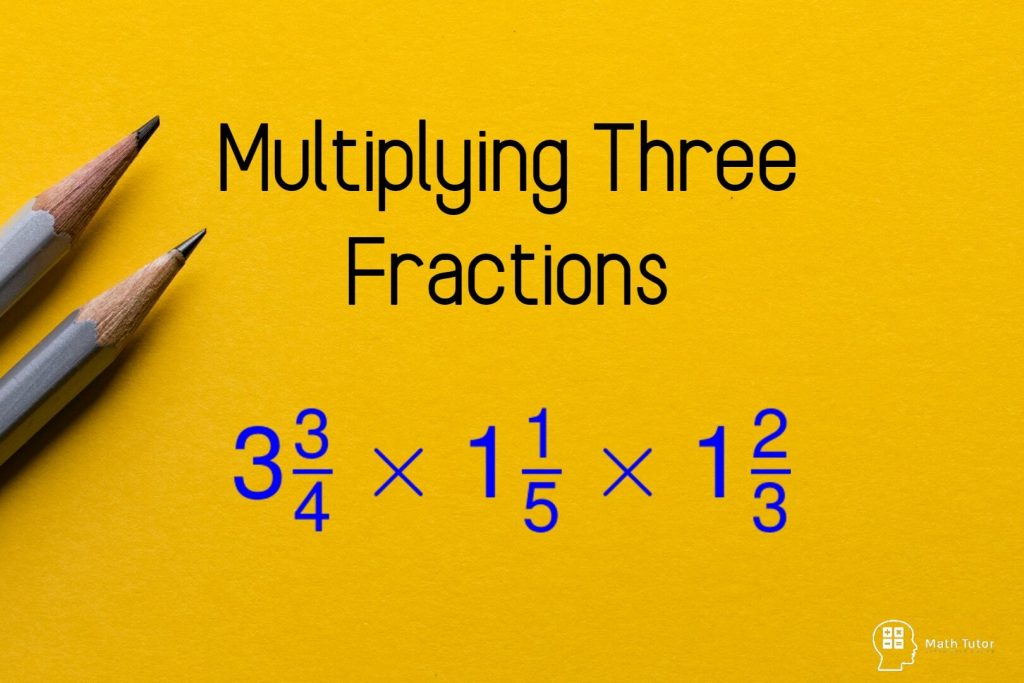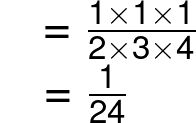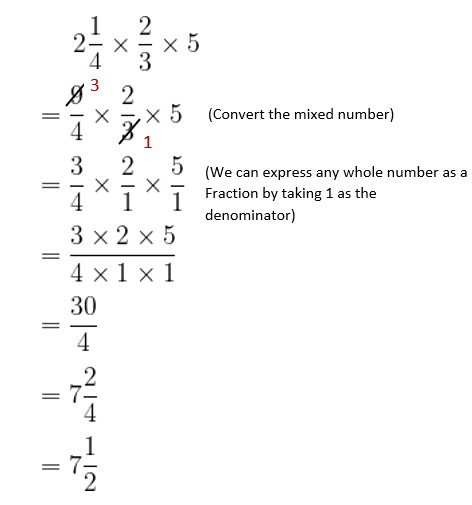# Multiplying Three Fractions

In this lesson, we are going to learn how to multiplying three fractions.
We have discussed how to multiply fractions from basics in another lesson. You can visit the following link to view the previous lesson.
How to Multiply Fractions

## Multiplying Three Fractions

Multiplying three fractions seems a little more complicated than multiplying two fractions. But the multiplication method is the same. We need to multiply the numerators and the denominators to find the product.
Before multiplying, simplify the fractions if you can. with that, you won’t end up with a large fraction to simplify at the end.

Example 01:; Multiply the numerators and the denominators separately.

Example 02:

In these types of problems before doing the multiplication it will make it easier if you can remove the common factors from the numerator.

In fractions we can remove common factors from the numerator and the denominator.

Let’s workout the same example in two ways.

01. First remove common factors.

02. First, multiply the numerators and the denominators the remove common factors in the answer.

Common factor for the numerator and denominator is 24.

Example 03:

Before doing any multiplications in this problem we have to convert the given mixed number into an improper fraction.

If it is difficult for you, you can refer to the lesson How to convert mixed numbers to improper fractions.

Now the answer is in the form of improper fraction. It is not suitable to keep your answer as an improper fraction. We need to convert it into a mixed number.

Related article: How to convert improper fractions to mixed numbers

Example 04:

Exercise:

## Multiply Following Three Fractions

You can always find these three fraction multiplication questions from the internet. Solve more questions and practice will make you better.
If you have any questions about the content of this article, feel free to drop a comment below.

Related Articles

### 5 thoughts on “Multiplying Three Fractions”

1.Great learning

2.One of your calculations is in correct – in ex 4 you have “1” by the crossed out “8”, it should be “2”.

1.Yes, You are correct. 🙂

3.What if one of the fraction is a whole number?

1.